# Consecutive numbers

Sum of ten consecutive numbers is 105. Determine these numbers (write first and last).

Result

a1 =  6
a10 =  15

#### Solution:Leave us a comment of example and its solution (i.e. if it is still somewhat unclear...):Be the first to comment!## Next similar examples:

1. Find the sumFind the sum of all natural numbers from 1 and 100, which are divisible by 2 or 5
2. SequenceBetween numbers 1 and 53 insert n members of the arithmetic sequence that its sum is 702.
3. AP - simpleDetermine the first nine elements of sequence if a10 = -1 and d = 4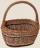Determine how many apples are in baskets when in the first basket are 4 apples, and in any other is 29 apples more than the previous, and we have eight baskets.
5. Students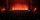In the front row sitting three students and in every other row 11 students more than the previous row. Determine how many students are in the room when the room is 9 lines, and determine how many students are in the seventh row.
6. AS sequenceIn an arithmetic sequence is given the difference d = -3 and a71 = 455. a) Determine the value of a62 b) Determine the sum of 71 members.
7. SequenceWrite the first 6 members of these sequence: a1 = 5 a2 = 7 an+2 = an+1 +2 an
8. SequenceWrite the first 7 members of an arithmetic sequence: a1=-3, d=6.
9. Sequence 2Write the first 5 members of an arithmetic sequence a11=-14, d=-1
10. Sequence 3Write the first 5 members of an arithmetic sequence: a4=-35, a11=-105.
11. Theorem proveWe want to prove the sentence: If the natural number n is divisible by six, then n is divisible by three. From what assumption we started?
12. SeatsSeats in the sport hall are organized so that each subsequent row has five more seats. First has 10 seats. How many seats are: a) in the eighth row b) in the eighteenth row
13. Sum of membersWhat is the sum of the first two members of the aritmetic progression if d = -4.3 and a3 = 7.5?
14. Saving per cents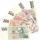The first day I save 1 cent and every next day cent more. How many I saved per year (365 days)?
15. Difference AP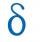Calculate the difference of arithmetic progression if the sum of its first 19 members Sn = 8075 and the first member is a1 = 20
16. Cans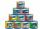How many cans must be put in the bottom row if we want 182 cans arrange in 13 rows above so that each subsequent row has always been one tin less? How many cans will be in the top row?
17. Series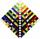Your task is express the sum of the following arithmetic series for n = 14: S(n) = 11 + 13 + 15 + 17 + ... + 2n+9 + 2n+11